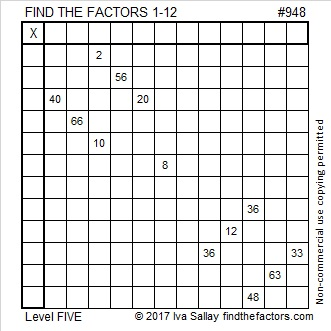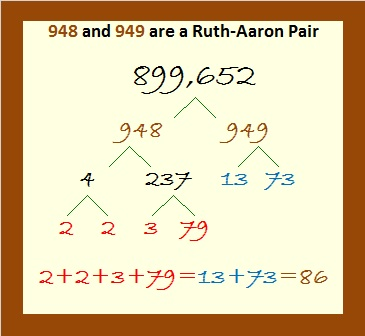# 948 and Level 5

All you need is a little bit of logic and the multiplication facts in a standard 12 x 12 multiplication table to solve this puzzle. This puzzle is not just for kids. It can be challenging even for adults. Go ahead, give it a try. You’ll soon see that it’s tougher than it looks, but stick with it, you can conquer it!Print the puzzles or type the solution in this excel file: 12 factors 942-950

Here’s something cool about the number 948 that I learned from OEIS.org:

948 and 949 make a Ruth-Aaron pair because they are consecutive numbers and the sum of 948’s prime factors equals the sum of 949’s prime factors:• 948 is a composite number.
• Prime factorization: 948 = 2 × 2 × 3 × 79, which can be written 948 = 2² × 3 × 79
• The exponents in the prime factorization are 2, 1, and 1. Adding one to each and multiplying we get (2 + 1)(1 + 1)(1 + 1) = 3 × 2 × 2 = 12. Therefore 948 has exactly 12 factors.
• Factors of 948: 1, 2, 3, 4, 6, 12, 79, 158, 237, 316, 474, 948
• Factor pairs: 948 = 1 × 948, 2 × 474, 3 × 316, 4 × 237, 6 × 158, or 12 × 79,
• Taking the factor pair with the largest square number factor, we get √948 = (√4)(√237) = 2√237 ≈ 30.7896This site uses Akismet to reduce spam. Learn how your comment data is processed.